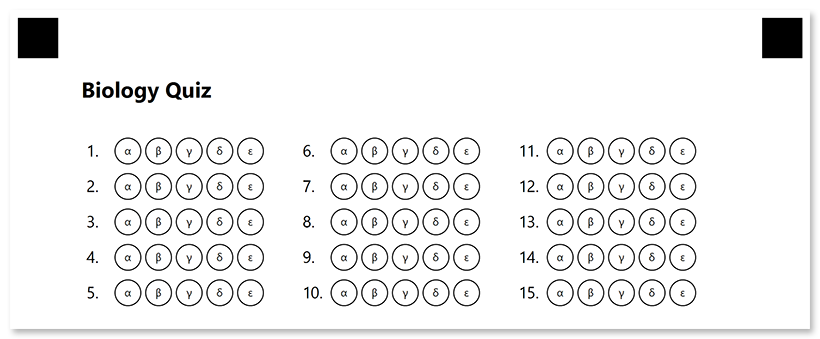Contents
[ ]

This element generates a numbered matrix of bubbles representing answers to a list of questions. Bubbles can be arranged in multiple columns to make more efficient use of space.

Answer_sheet is best suited for exam papers where you want to fit the maximum number of answers on a single page.

## Syntax

The element is declared with `?answer_sheet=[name]` statement. This statement must be placed on a separate line.

`name` property is used as an element’s identifier in recognition results and as a reminder of the element’s purpose in template source; for example, “Biology Quiz”. The name is not displayed on the form.

### Attributes

An attribute is written as `[attribute_name]=[value]`. Each attribute must be placed on a new line immediately after the opening `?answer_sheet=` statement or another attribute, and must begin with a tab character.

#### Required

Specify the number of exam questions that the answer sheet corresponds to in the elements_count attribute. Each question will correspond to a numbered line with multiple answer bubbles.

For example, `elements_count=100`.

#### Optional

The answer_sheet element can be customized by adding optional attributes to it.

Attribute Default value Description Usage example
columns_count 4 The number of columns to arrange lines into. Use multiple columns to make the answer sheet more compact. `columns_count=3`
You can only set the same number of answers for all questions. If the number of answers is different for each section of the exam, use multiple answer_sheet elements.
`answers_count=5`
start_id Automatic The number of the first line used as a base for further numbering.
If omitted, the number will be calculated based on the numbering of previous elements.
`start_id=1`
vertical_margin 0 Vertical spacing between lines, in pixels. `vertical_margin=10`
bubble_size Normal Size of bubbles: `extrasmall`, `small`, `normal`, `large`, or `extralarge`. `bubble_size=large`
bubble_type round Bubble style: `round` or `square`. `bubble_type=square`
answers_list A to Z Characters to be drawn inside bubbles in ({Character 1})({Character 2})…({Character N}) format.
The total number of characters must match the value of the answers_count attribute.
`answers_list=(α)(β)(γ)(δ)(ε)`
column 1 The number of the column where the answer_sheet element will be placed. Only applicable if answer_sheet is placed in a multi-column container. `column=2`

None.

## Example

``````?text=Biology Quiz
font_size=16
font_style=bold
?empty_line=